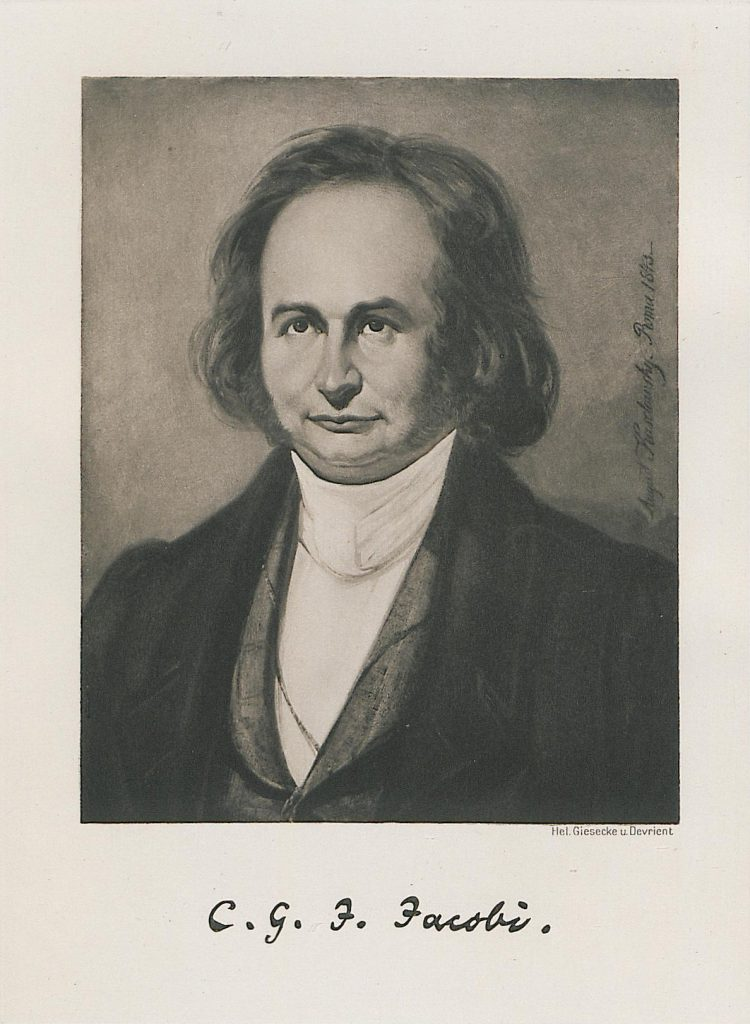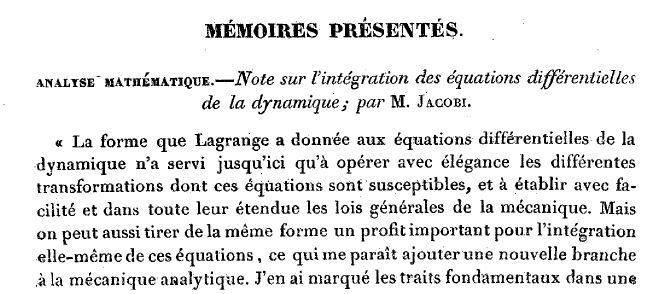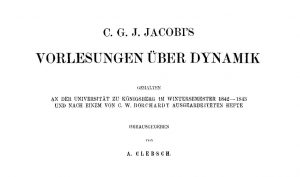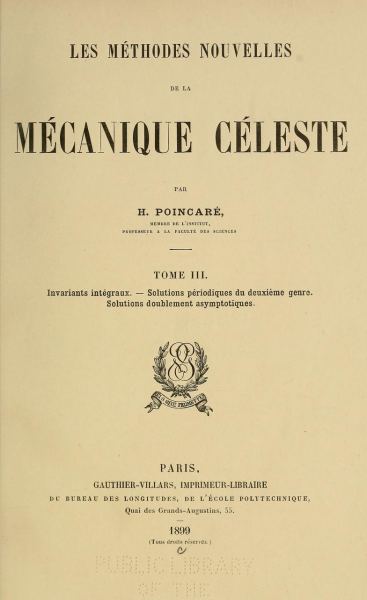CSHPM Notes bring scholarly work on the history and philosophy of mathematics to the broader mathematics community. Authors are members of the Canadian Society for History and Philosophy of Mathematics (CSHPM). Comments and suggestions are welcome; they may be directed to either of the column’s co-editors:

Amy Ackerberg-HastingsIndependent Scholar (aackerbe@verizon.net)
Hardy GrantYork University [retired] (hardygrant@yahoo.com)

The authors met at a conference on the history of mathematics that was held in the summer of 1990 in Tokyo. Both of us worked on the history of analysis and mechanics, with Fraser focusing more on the 18th century and Nakane on the 19th century. We had a common interest in the intellectual or technical development of science with a particular focus on the interplay between mathematics and physics. This common interest led over the following years to a collaboration that resulted in 2003 in an article on the early history of Hamilton-Jacobi theory .

The present project developed from a survey that we also published in 2003, in an Italian encyclopedia, on mathematical methods in 19th-century celestial mechanics . What was initially a plan to write an English version of this essay evolved over the years into a rather different project centered on the history of the concept of canonical transformation. We discussed our research at the International Congresses of the History of Science held in 2009 in Budapest and in 2017 in Rio de Janeiro, and corresponded occasionally in the years between. The research really got underway in earnest early in 2018, and the collaboration has involved many emails and multiple drafts exchanged since then between Toronto and Tokyo. The authors presented some of the preliminary results of their research at the joint meeting of the AMS and MAA in Denver in January 2020. Talks have also been given at the annual meeting of the CSHPM (Vancouver 2019) and the winter meetings of the CMS (Montreal 2015 and online 2020). The projected date of publication of the study is later in 2021 or early 2022.Figure 1. Carl Gustav Jacobi (1804-1851) in 1843. Public domain.

Hamilton-Jacobi theory is composed of three major parts: the canonical ordinary differential equations, the Hamilton-Jacobi partial differential equation, and the concept of canonical transformation. The first two elements were established by William Rowan Hamilton and Carl Gustav Jacobi in the 1830s. The concept of a canonical transformation was introduced by Jacobi in 1837 , but really only became a central part of the theory more than fifty years later. The present research project follows the original formulation and subsequent development of Jacobi’s results on transformations until about 1950.

Consider the 2n variables $q_{i}=q_{i}\left(t\right)$ and $p_{i}=p_{i}\left(t\right)$ (i=1,…, n) where the time t is the independent variable. The Hamiltonian H is a function of t, qi and pi:

$$H=H \left( t, q_{i},p_{i} \right)~~~~~\left(1\right)$$

The system is governed by Hamilton’s equations for the problem:

$$\begin{matrix}\frac{dp_{i}}{dt}=-\frac{ \partial H}{ \partial q_{i}},\\\frac{dq_{i}}{dt}=\frac{ \partial H}{ \partial p_{i}}.\\\end{matrix}~~~~~~~~~~~~\left(2\right)$$

(2) are also known as the “canonical equations,” terminology that originated with Jacobi.

Suppose now that we transform $q_{i}$ and $p_{i}$ to new variables $Q_{i}$ and $P_{i}$:

$$q_{i}=q_{i} \left( Q_{i},P_{i} \right) ,~p_{i}=p_{i} \left( Q_{i},P_{i} \right)~~~~~~~\left(3\right)$$
The Hamiltonian then becomes a function of t, $( Q_{i} )$ and $( P_{i} )$, $H=H \left( t, Q_{i},P_{i} \right)$ . Suppose the given transformation has the property that equations (2) transform to the equations
$$\begin{matrix}\frac{dP_{i}}{dt}=-\frac{ \partial H}{ \partial Q_{i}},\\\frac{dQ_{i}}{dt}=\frac{ \partial H}{ \partial P_{i}}.\\\end{matrix}~~~~~~~~~~~~\left(4\right)$$

which are canonical in the variables $Q_{i}$ and $P_{i}$. A transformation with such a property is said to be “canonical.” The goal is to transform to variables that are integrable and thereby obtain a solution to the problem.

Consider any function $\psi = \psi(q_{i},Q_{i})$ of $q_{i}$ and $Q_{i}$. $\psi$ is what is called a “generating function” in modern textbooks. We require that the variables $q_{i}$, $p_{i}$ and $Q_{i}$$P_{i}$ satisfy the equations:
$$p_{i}=\frac{ \partial \psi }{ \partial q_{i}},~P_{i}=-\frac{ \partial \psi }{ \partial Q_{i}}~~~~~~ \left(5\right)$$

(5) serves to define a transformation between the old and new variables, expressing $q_{i}$ and $p_{i}$ as functions of $Q_{i}$ and $P_{i}$. For a transformation defined in this way equations (4) hold and the transformation is canonical. This is Jacobi’s fundamental theorem on canonical transformations.

As noted above, Jacobi stated this result in a note published in 1837 in the Comptes rendus of the Paris Academy of Sciences. He provided no proof but somewhat misleadingly suggested it is not difficult to give one. Jacobi died in 1851, but a detailed proof of the theorem was only published in an appendix to his famous 1866 lectures on dynamics . Jacobi is believed to have written this essay in the late 1830s, although the exact dating is uncertain. The 1866 lectures themselves were originally delivered in the winter semester of 1842–43 at the University of Königsberg. Jacobi’s proof (generalized to include time-dependent generating functions) was adopted by the Swedish astronomer Carl Charlier in volume two (1907) of his influential treatise on celestial mechanics . (When the generating function $\psi=\psi(t, q_{i}, Q_{i})$ is also a function of time the transformation equations (3) involve the time and H is replaced by $K=H+\frac{ \partial \psi }{ \partial t}$ in (4).)Figure 3. Writing in French was unusual for him.Figure 4. Portions of the title page of Jacobi's lecture, published in German in 1866 and read much more widely in the late 19th century than his 1837 paper.

In articles that fell into historical obscurity, proofs of Jacobi’s theorem on canonical transformations were published by two mathematical astronomers, Adolphe Desboves in 1848 and William Donkin in 1854 [4; 5]. However, the concept of a such a transformation really only became a central part of the theory in the 1890s in the writings of Henri Poincaré, in successive editions of his lectures on celestial mechanics. In particular, Poincaré in 1899 presented what would become the standard proof of Jacobi’s result that one finds in all textbooks today . This demonstration deploys a variational principle to produce an elegant structural proof that is much simpler than the derivations of Jacobi, Desboves and Donkin. It was also Poincaré and his contemporary Edmund Whittaker who hit upon the idea of using a solution of the Hamilton-Jacobi partial differential equation as the generating function for a canonical transformation .Figure 6. Title page for the 1899 edition of Poincaré's lectures on celestial dynamics.

From the beginning various forms of the three-body problem in astronomy were the primary physical focus of Hamilton-Jacobi theory. In the second decade of the 20th century the methods of Hamilton, Jacobi, Poincaré and Charlier were adopted by German quantum physicists and became central to the new theories of matter that they were constructing. One might say that these events constitute a striking example of the unreasonable effectiveness of mathematics in natural science. In 1919 physicist Arnold Sommerfeld wrote

Up to a few years ago it was possible to consider that the methods of mechanics of Hamilton and Jacobi could be dispensed with for physics and to regard it as serving only the requirements of the calculus of astronomic [sic] perturbations and the interests of mathematics.

Sommerfeld draws attention to the key place these methods had come to occupy in physics, observing that

it seems almost as if Hamilton’s method was expressly created for treating the most important problems of physical mechanics .

An exposition of Hamilton-Jacobi theory was given by Max Born in 1925 in his famous book on atomic physics . Two years later Lothar Nordheim (a student of Born and an assistant of David Hilbert) and Erwin Fues composed a critical distillation of the theory for the Handbuch der Physik . Their account became the source for such standard textbook accounts as Herbert Goldstein’s Classical Mechanics (1950) in physics  and Israel Gelfand and Sergei Fomin’s Calculus of Variations (1963) in mathematics . The subject of canonical transformations was also explored in a somewhat more abstract mathematical form by Constantin Carathéodory in his authoritative 1935 book on analysis .

Poincaré was familiar in a general way with some of the standard results in dynamical analysis, particularly as these had been presented in writings of astronomers such as Félix Tisserand on perturbations. However, he wrote with a limited historical sense for the work of his predecessors in mechanical science. Research that became standard in the 20th century took off from Poincaré and Charlier and was even less rooted in the older historical sources. One of the challenges of our study has been to identify, understand and document the byways of 19th-century research in analytical dynamics and analysis. The interplay of celestial mechanics and mathematical subjects such as the calculus of variations and theory of partial differential equations was a fascinating arena for some of the most advanced natural science of the period.

Craig Fraser teaches the history of mathematics and astronomy at the University of Toronto. He is President of the Canadian Society for History and Philosophy of Mathematics and a full member of the International Academy of the History of Science. He recently published “Mathematics in Library and Review Classification Systems: An Historical Overview” in Knowledge Organization 47, no. 4 (2020), 334–356.

Michiyo Nakane is a part-time lecturer at Seijo University in Tokyo. An historian of the mathematical sciences, she investigates the development of theoretical mechanics and celestial mechanics in the 19th and early 20th centuries. She is currently studying the reception and development in Japan of mathematical methods of quantum physics. She recently published “Yoshikatsu Sugiura’s Contribution to the Development of Quantum Physics in Japan” in Berichte zur Wissenschaftsgeschichte 42 (2019), 338–356.

### References

 Born, Max. (1925) Vorlesungen über Atommechanik. With the assistance of Friedrich Hund. Berlin: Springer.

 Carathéodory, Constantin. (1935) Variationsrechnung und partielle Differentialgleichungen erster Ordnung. Leipzig and Berlin: B. G. Teubner.

 Charlier, Carl Ludwig. (1907) Die Mechanik des Himmels. Vol. 2. Leipzig: Veit & Comp. Jacobi’s transformation theorem is given in the 11th section.

 Desboves, Adophe H. (1848) Démonstration de deux théorèmes de M. Jacobi – Application au problème des perturbations planétaires. Journal de mathématiques pures et appliquées 13, 397–411. Thesis presented to the Paris Faculty of Sciences, 3 April 1848.

 Donkin, William F. (1855) On a class of differential equations, including those which occur in dynamical problems – Part II. Philosophical Transactions of the Royal Society of London 145, 299-358.

 Fraser, Craig, and Michiyo Nakane. (2003) La meccanica celeste dopo Laplace: la teoria di Hamilton-Jacobi. In Storia della scienza, editor-in-chief Sandro Petruccioli, vol. 7, 234–243. Roma: Istituto della Enciclopedia Italiana.

 Gelfand, Israel M., and Sergei V. Fomin. (1963) Calculus of Variations. Revised and translated by Richard A. Silverman. Englewood Cliffs, NJ: Prentice-Hall Inc.

 Goldstein, Herbert. (1950) Classical Mechanics. Reading, MA: Addison-Wesley Publishing Company, Inc.

 Jacobi, Carl Gustav J. (1837) Note sur l’intégration des équations différentielles de la dynamique. Comptes rendus de l’Académie des Sciences de Paris 5, 61–67. Reprinted in Jacobi’s Gesammelte Werke, vol. 4 (1886), 129–136.

 Jacobi, Carl Gustav J. (1866) Über diejenigen Probleme der Mechanik, in welchem eine Kräftefunction existiert und über die Theorie der Störungen. Supplement to Vorlesungen über Dynamik (1866, 303–470). Reprinted with minor editorial emendations in Jacobi’s Gesammelte Werke, vol. 5 (1890), 217–395.

 Nakane, Michiyo, and Craig Fraser. (2003) The Early History of Hamilton-Jacobi Theory. Centaurus 44, 161–227.

 Nordheim, Lothar, and Erwin Fues. (1927) Die Hamilton-Jacobische Theorie der Dynamique. In Handbuch der Physik, edited by Hans Geiger and Karl Scheel, vol. 5, 91­–130. Berlin: Springer.

 Poincaré, Henri. (1899) Les Méthodes nouvelles de la mécanique céleste. Vol. 3. Paris: Gauthier-Villars.

 Sommerfeld, Arnold. (1919) Atombau und Spektrallinien. Braunschweig: Wieweg & Sohn. The English translation by Henry L. Brose published in 1923 is of the German third edition, also published in 1923. The passages quoted above are the same in the different German editions and appear as quoted on pp. 555–556 of the English translation.

 Whittaker, Edmund Taylor. (1904) A Treatise on the Analytical Dynamics of Particles and Rigid Bodies: With an Introduction to the Problem of Three Bodies. Cambridge: Cambridge University Press.